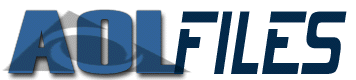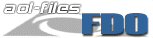AOL-FilesArticlesDownloadsFDOAtomsTokensOriginal FDOAOL AreasAMPsFDO Tutorial: Lesson 3 - Buttons by Tau This lesson will show how buttons can be placed on forms along with and how you can use atom properties to customize the buttons. The form we will be using throught this lesson is the one that was made in the last lesson. The code is shown below. ```uni_start_stream man_start_object mat_orientation mat_position mat_bool_resize_vertical mat_precise_width <300> mat_precise_height <150> mat_bool_precise mat_art_id <1-1-40898> mat_bool_background_pic man_end_object man_update_display uni_end_stream ``` Adding a Button To place the first object on the form you add the following atom after the form properties: man_start_object Note that this is only for one object on the form, additional objects require a different atom. So to add a command button to this form with the caption of "Test" the following code would be used: ```uni_start_stream man_start_object mat_orientation mat_position mat_bool_resize_vertical mat_precise_width <300> mat_precise_height <150> mat_bool_precise mat_art_id <1-1-40898> mat_bool_background_pic man_start_object man_end_object man_update_display uni_end_stream ``` Note that "trigger" is the class name for buttons. This alone will not do anything though. It would be like drawing a picture without knowing what to draw, what size, shapes, colors, and everything else. So the properties need to be added. Setting the Properties Properties for the button follow the man_start_object and for easy reading it is indented one more space. mat_bool_default - Determines if the button is default. If it is, then when enter is pressed on the form the button will be activated. mat_trigger_style - Makes the button a rectangle. mat_font_sis - This determines the properties of the caption of the button. Font is the font that the button will have, size is the size of the caption text, and attributes can be normal, bold, italic, or underline mat_color_face - Sets the background color of the button in RGB format. Values can be 0 to 255 for each. RGB values can be found here. mat_color_text - Sets the color of the caption in RGB format. Values can be 0 to 255 for each. RGB values can be found here. mat_color_top_edge - Sets the color of the top edge in RGB format. Values can be 0 to 255 for each. RGB values can be found here. mat_color_bottom_edge - Sets the color of the bottom edge in RGB format. Values can be 0 to 255 for each. RGB values can be found here. mat_horizontal_spacing <#> - Sets the spacing between the button and the object above it. mat_precise_width <#> - Sets the width of the button in pixels. mat_precise_height <#> - Sets the height of the button in pixels. mat_precise_x <#> - Sets the vertical distance from the top of the form that the bottom will be. mat_precise_y <#> - Sets the horizontal distance from the left of the form that the button will be. mat_art_hint_title <"caption"> - This is a preview of the button that will appear while it is loading. It will only be viewable for a short amount of time, if at all depending on how quickly the form loads mat_art_id - This will set the picture of the button. id is the art record of the picture. mat_font_size <#> - Sets the font size of the button if mat_font_sis is not being used. mat_context_help <"caption"> - Sets the text seen when the mouse is moved over the button. mat_relative_tag <#> - Sets the buttons relative ID which will be explained in a later lesson. The following code is for a single button to be placed in the bottom right corner of the form. Using the properties above and with some of your own testing you can easily master designing buttons. Quite a talent, eh? ```uni_start_stream man_start_object mat_orientation mat_position mat_bool_resize_vertical mat_precise_width <300> mat_precise_height <150> mat_bool_precise mat_art_id <1-1-40898> mat_bool_background_pic man_start_object mat_context_help <"Click here!"> mat_trigger_style mat_bool_precise mat_precise_width <70> mat_precise_height <24> mat_precise_x <220> mat_precise_y <120> mat_font_sis mat_color_face <0, 109, 170> mat_color_text <255, 255, 255> mat_color_top_edge <145, 182, 255> mat_color_bottom_edge <1, 1, 1> man_end_object man_end_object man_update_display uni_end_stream ``` This will result in:mat_end_object is placed after all the properties of the button to show that you are done setting its properties. Infinite amount of designs can be made with this code.While designing your own forms you can look at the Atomc Debugger FDO stream. Search for "trigger" in the FDO stream and look at the code for other buttons. Most are made using the properties above. Actions What fun is a button that cannot do anthing? Add the following code after the last property of the button: ```act_replace_select_action < uni_start_stream code uni_end_stream > ``` code can be many things. For example, if you want to make a message box saying, "Hey! This is my first button test!" then you could replace code with: async_alert Another simple thing you do is send token arguments. Replace code with: sm_send_token_arg <"L1", 28> and it would take you to the Enter Private Chat form. Putting it all together ```uni_start_stream man_start_object mat_orientation mat_position mat_bool_resize_vertical mat_precise_width <300> mat_precise_height <150> mat_bool_precise mat_art_id <1-1-40898> mat_bool_background_pic man_start_object mat_context_help <"Click here!"> mat_trigger_style mat_bool_precise mat_precise_width <70> mat_precise_height <24> mat_precise_x <220> mat_precise_y <120> mat_font_sis mat_color_face <0, 109, 170> mat_color_text <255, 255, 255> mat_color_top_edge <145, 182, 255> mat_color_bottom_edge <1, 1, 1> act_replace_select_action < uni_start_stream sm_send_token_arg <"L1", 28> uni_end_stream > man_end_object man_end_object man_update_display ``` Simple, right? Well mess with it, you'll get it eventually. ;)
 © 2000 BMB and Tau Productions. Contacts: BMB | Tau | Rob | Mage